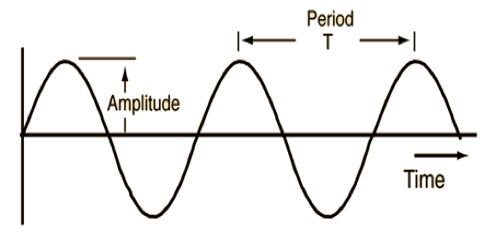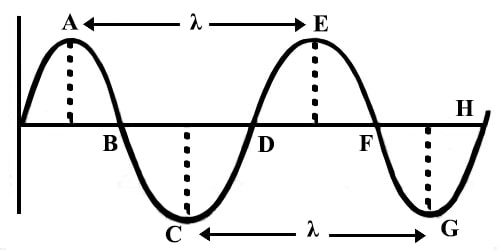Physics

# Time Period of WaveTime Period of Wave

The time required by a particle of the medium to execute one complete oscillation is called the time period of the wave. It is denoted by T. Let the time taken by a particle or source to complete N number of oscillation be t, then time period T = t/N. In Fig., the time is taken by a wave to travel the distance AE (wavelength denoted by λ) is the time period T. As the frequency of a wave increases, the time period of the wave decreases.Fig: Time period of Wave

A wave is an example of periodic motion. It refers to a particular time in which a work is completed but when it is repeatedly, and then we say that particular task is periodical manner. So the wave period is equal to the time in medium’s particle completes one complete vibrational cycle. But frequency shows to how much time something has happened. The particles of the medium oscillate back and forth about a fixed position. The time which it takes for such a particle to complete one full cycle is known as the period. Period units are simply seconds (or minutes or hours or some time unit). The period of a wave is the time for a particle on a medium to make one complete vibrational cycle. Period, being a time is measured in units of time such as seconds, hours, days or years.